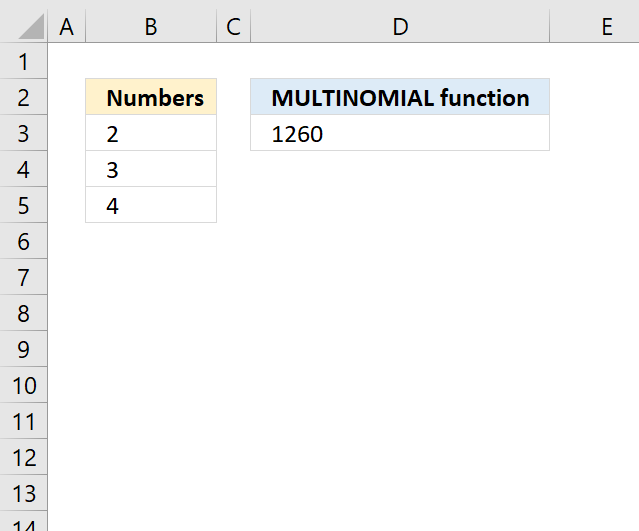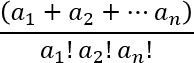Author: Oscar Cronquist Article last updated on April 23, 2018The MULTINOMIAL function calculates the ratio of the factorial of a sum of values to the product of factorials.

Formula in cell D3:

=MULTINOMIAL(B3:B5)

The value is calculated like this:

=FACT(2+3+4)/(FACT(2)*FACT(3)*FACT(4))

See mathematical formula below in comments.

### Excel Function Syntax

MULTINOMIAL(number1, [number2], ...)

### Arguments

 number1 Required. The number for which you want to calculate the multinomial. [number2] Optional. Up to 254 additional numbers.